# Red-Black Trees

• Red Black Trees asre binary search trees where all nodes in the tree have an extra property: color. The nodes can be either red, black, or (occasionally) double black.
• The trees have the following properties:
1. Root is black
2. All the external nodes are dummy nodes with no elements, and they are colored black.
3. The children of red nodes are always black.
4. All external nodes have the same black depth, where black depth is defined as the number of black ancestors of that node.
• The following is an example red black tree.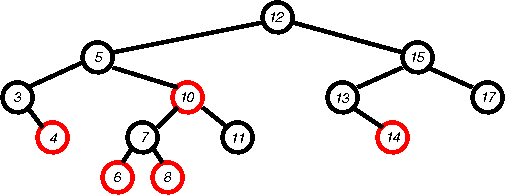• Proposition: The height of a red-black tree is O(log n)
1. The black depth of the tree is O(log nb), where nb is the number of black nodes. To see this:
1. imagine we removed all the red nodes- this would not change the black depth, but now the black depth of the leaves is the same as the height (no red nodes, see?).
2. Thus we have a tree where all leaves have the same depth, or a complete tree.
3. We know that complete trees have height O(log n).
4. So, since the black depth of the original tree is equal to the height of the modified tree, and the height of the modified tree is O(log nb), the black depth of the original tree is O(log nb).
2. In the worst case, that is the case with the tallest tree, there must be some long path from the root to a leaf.
3. Since the number of black nodes on that long path is limited to O(log nb), the only way to make it longer is to have lots of red nodes.
4. Since red nodes cannot have red childred, in the worst case, the number of nodes on that path must alternate red/black.
5. thus, that path can be only twice as long as the black depth of the tree.
6. Therefore, the worst case height of the tree is O(2 log nb).
7. Even if the tree is filled with red nodes, nb is O(n) because at most, roughly half the tree will be red. [whoa, that's a big handwave]
8. Therefore, the height of a red-black tree is O(log n).
• There is an important correspondence between red-black trees and 2-3-4 trees. A black node and its red children are equivalent to a single node in a 2-3-4 tree.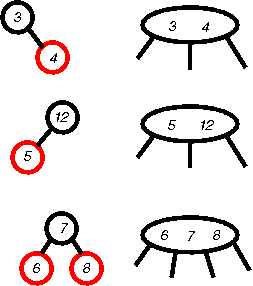A black node with black children is just a 2 node in a 2-3-4 tree. We will use this correspondence to make sense of things later on.

## Insertion

• Now that we know about red-black trees, what do we do with them? Well, we insert and delete things. Let's talk about how insert works.
1. The first step is just like with regular BSTs, find an empty spot at the leaves that would maintain the search tree order, and insert a new node.
2. Since adding the new node as a black node would change the black depth of the leaves along that path, we make the new node red.
3. This may create two consecutive reds, which requires fixin'. Fixin is done with rotations and recolorings. We'll justify these by refering back to 2-3-4 trees.
1. What if we add a node z that is a child of a red node v which is a child of a black node u (u can't be red or the tree wouldn't have been a red black tree in the first place) and u's other child w is a black node? One configuration is like: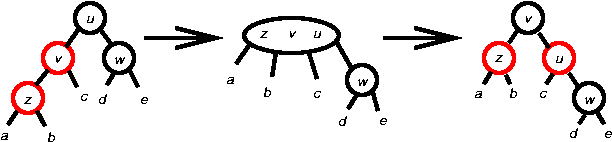The a,b,c,d,e all represent the sub-trees beneath these nodes. When the node z is first inserted, all these sub-trees are just the dummy leaf nodes. Node w is also a dummy leaf node. As we will see later on, we may need to apply this rule at another time when the node we're refering to a z was not the just inserted node. stay tuned.

Since this case described here corresponds to the shown 2-3-4 tree, all we need to do is convert the color and structure of the local red-black nodes so that they represent the same 2-3-4 structure, but without the double red. That is just what is done above.

2. Another configuration of the same situation shown here has a similar solution: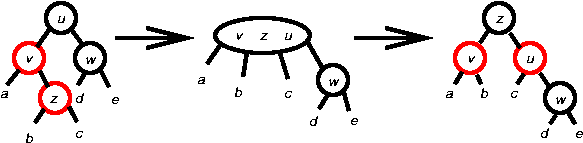3. What if that node w was red?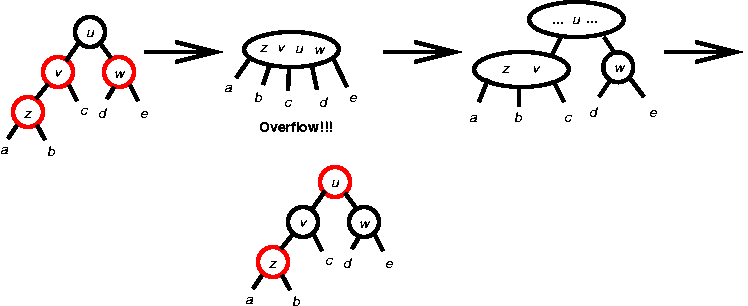But note, just as in the 2-3-4 case, where passing up the u to the parent could cause another overflow, making u red could cause another consecutive red problem. Fortunately, we now know how to solve consecutive reds- by applying these transformations (this is why a,b,c,d,e could be internal nodes: we're applying the rules higher up in the tree because the double red cascaded up.

4. What if the double red cascades all the way up to the root? In that case, we re-color the root black. This increases the black depth of every single leaf by one. This is equivalent to adding a new node at the root in a 2-3-4 tree.
• How long does the insert take?
1. To find the place to insert takes the height of the tree, or O(log n).
2. To add the node is O(1).
3. To fix possible double reds, a rotation is O(1).
4. Worst case, the double red can cascade all the way to the root. The cascade is proportional to the height of the tree, so the fixin' takes O(log n), worst case.
5. Therefore, insertion is O(log n).

## Deletion

• The other thing we'll probably do with our red-black tree is delete things from it.
1. The first thing is to find the node to delete. This is the same as any BST.
2. Next, we'll want to move the element to delete down to a node that's easier to get rid of. Just as with BSTs, we'll find the left-most right descendant and swap contents.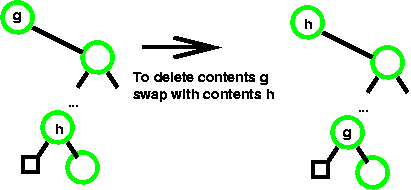We'll use the color green to indicate that we either don't know or don't care what the colors are. Note that we're swaping the contents, not the node, so the colors stay where they are.

3. We remove the node with the contents we want to delete, and move up that node's right child, just as with BSTs.
4. If the node deleted was red, then there's nothing left to do: We didn't affect the black depth, and since the tree was already a valid red-black tree with no consecutive reds, there will still be no consecutive reds.
5. If the node we deleted (we'll call it v) was black, we've broken the black depth for its descendants and possible created consecutive reds. This must be fixed:
1. If the child of v (we'll call it r) was red, then there's no problem: we color r black and all is fixed: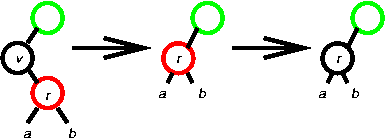2. If r is black, we can't change it from red to balck to preserve black depth. Instead we'll color extra-black (really really black?) which we'll call double-black. Double black will count two towards the black depth on that path. But double-black is not really a valid color for a node so we have to fix that. Yes, I'm aware this sounds really stupid, but it actually has a point: by keeping a node double-black, we know exactly where the black shortage is. Otherwise it would be easy to lose this shortage in the greater tree. It's a placeholder for us.
3. What we do about the double black depends on the colors of the neightboring nodes (after v is removed). For instance, if r's sibling is black with a red child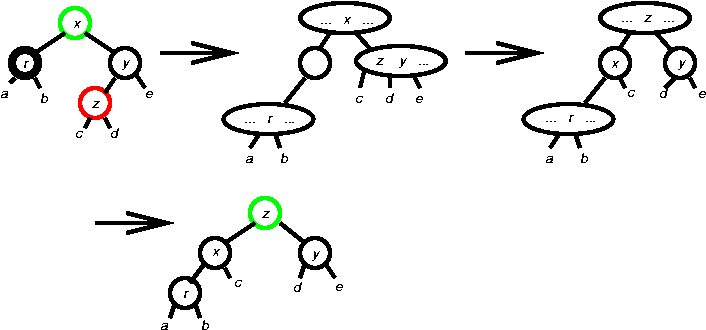The double black corresponds to an underflow node in a 2-3-4 tree. In the final tree, the color of z is the same as the color of x in the initial tree.

If the red child of y was on the other side, we get: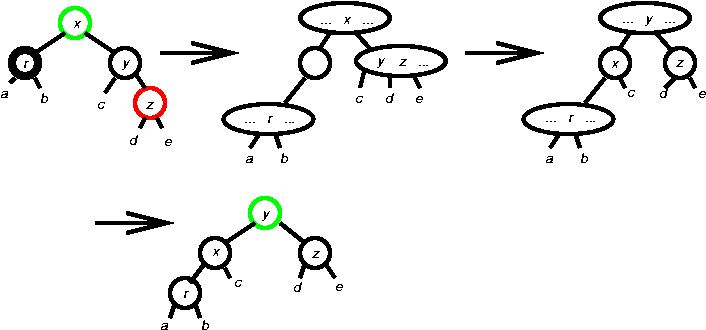4. if v was black, r is black, y is black and y has no red children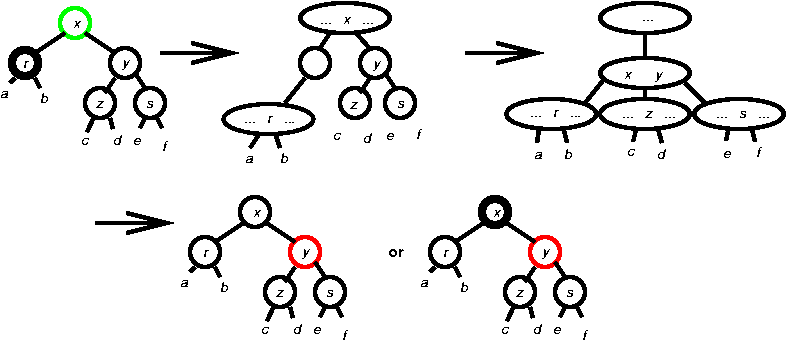This corresponds to an underflow in a 2-3-4 tree where there are no elements in the sibling in that case to borrow from. Instead, an element is dropped from the parent node, and the two nodes are merged. Dropping from the parent could cause an underflow in the parent which may propagate up the tree. In the case of the red-black tree, if x was red, then coloring it black is equivalent to dropping it, and making y red is equivalent to merging. If x was already black, it was already a 2-node, and thus dropping it would cause another underflow in the 2-3-4 tree, so we must color x double-black to indicate we still have an underflow.

5. In this manner, the double-black can be passed up the tree the same way underflow can be.
6. If double-black reaches the root, then it can be made a single black, removing one from the black depth of every single leaf node.
7. The final case is when v was black, r is black, and y is red. In this case we just rotate the tree to an equivalent position, but so that r's sibling (which used to be y) is now black. Then we apply one of the previous rules.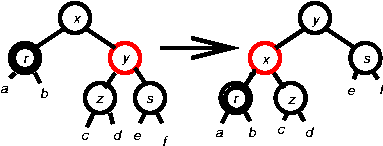6. How long does the deletion take?
1. Finding the node to delete plus finding the left-most right descendant is proportional to the height of the tree, so it is O(log n).
2. The swaping and deletion is O(1).
3. Each individual fix (rotation, etc.) is O(1).
4. In the worst case, a double-black may get passed up to the root. Since each rotation takes constant time, this would be proportional to the height of the tree, and thus is O(log n).
5. Therefore, the worst case cost of deletion is O(log n).